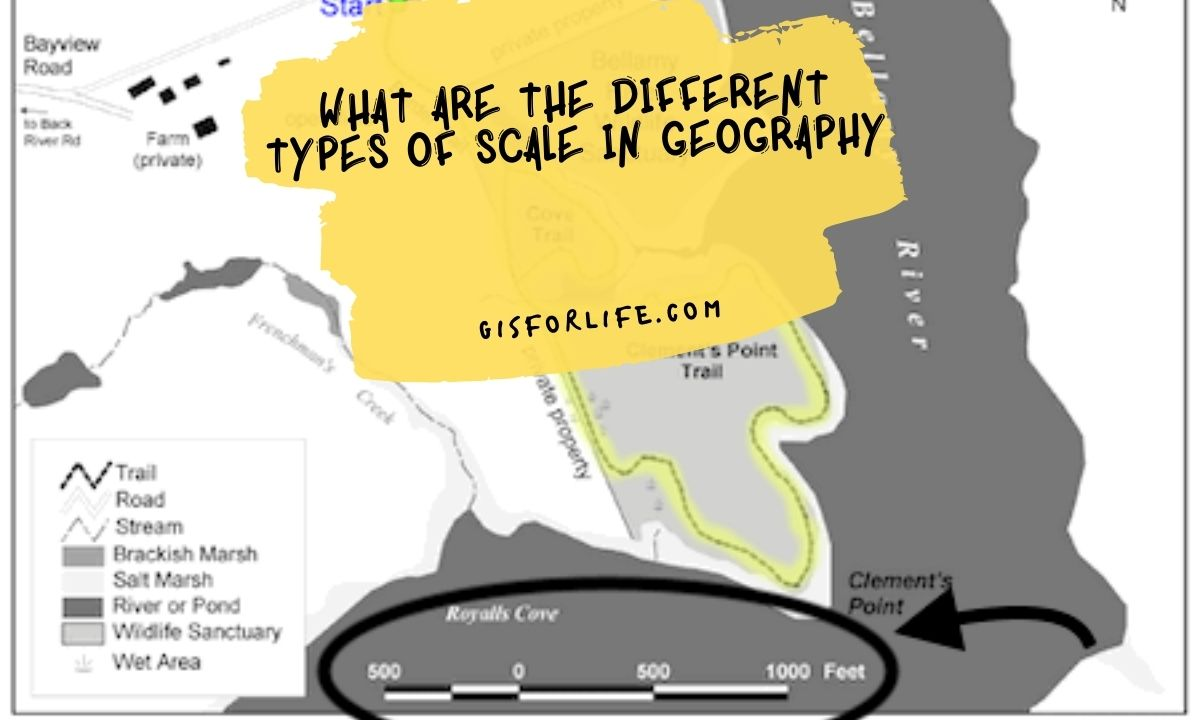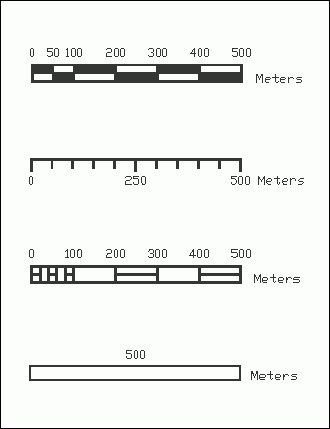## What Are the Different Types of Scale in GeographyDifferent types of scales are used to define geographical objects. A line can be drawn on a map to show the shortest distance between two points, for example. Different types of scales are used to measure distance on maps. Direct measurement is obtained on the globe’s surface, while distance on the map is measured by counting the number of grid squares between two points. This is known as a ‘distance’ scale.

There are three types of scales used on maps: linear, angular, and areal. The linear scale is measured in meters or kilometers and corresponds to the distance on the ground. Angular scale measures angle in degrees and is used to calculate distances on a map. Areal scale is the ratio of the area on the map to the actual area it represents, using square kilometers or square meters as a unit.

## Things to know when creating meaningful scales

Scales can be broken down into 4 categories: Linear Scales, Angular Scales, Area Scales, and Hybrid Scales.

### Linear Scales

There are three types of linear scales: small, medium, and large.

A small-scale map will represent a country’s interstate highways while a large-scale map will show local roads and thoroughfares in addition to the interstate system. Maps that only display the interstate highway system belong to a medium scale.

Linear Scale Example: When you drive, the distance between two points can be measured on a map using a linear scale. By counting the number of grid squares between these points, an estimation of how far apart they are can be made. This is just a one-way linear scale that can be used to measure distance.

### Angular Scales

The two most common types of angular scales are a small- and a large-angle. A large angle represents a smaller area on the map while a small angle is used to represent a larger area. A small-scale map has a large angle, enabling greater detail. Conversely, large-scale results in less detail.

Angular Scale Example: If you want to find out how far away a mountain is from a town, the angular scale can be used. The angle between these two points can be measured and then converted into miles or kilometers. This will give you a better estimate of how far apart they are.

### Area Scales

The most common area scales are small, medium, and large. Small-scale maps do not represent broad areas or the entire geographical extent of an object. Medium-scale maps incorporate more information but remain relatively small in scale when compared to a large-scale map which is usually used for regional mapping. Large-area scales are not commonly used and when they are, the map covers a vast area.

Area Scales Example: Area scales provide an overview of large geographic areas and can give a person a general idea about what to expect when exploring or traveling through this area. For example, medium-scale maps display most urban environments while small-scale maps depict cities with greater detail such as contour lines and specific buildings.

### Hybrid Scales

A hybrid scale is a combination of two or more scales. The most common type of hybrid scale is the small-medium scale which combines the features of a small-scale map with the general overview of a medium-scale map. This type of scale is used to display both fine detail and wide geographic areas.

Hybrid Scale Example: A hybrid scale is a combination of two or more scales. For example, the small-medium map is very helpful in finding out the distance between points when looking at an area on a larger scale. This can be useful when planning road trips across long distances.

## Scales on Maps: Everything You Need to Know

Maps are an essential tool for navigation and orientation. They provide us with the necessary information to move around with ease and reach our desired destination. One of the most crucial aspects of a map is the scale, which represents the relationship between the distance on the map and the actual distance on the ground. Understanding map scales is crucial for accurate navigation, and in this article, we will cover everything you need to know about scales on maps.

### Types of Map Scales

There are three main types of map scales: ratio, verbal, and graphic. The ratio scale is represented as a fraction, such as 1:50,000, where one unit on the map represents 50,000 units on the ground. Verbal scales are represented as a statement, such as “one inch equals one mile.” Graphic scales are represented as a line with markings that correspond to actual distances on the ground.

### Understanding Map ScaleUnderstanding map scale is essential for accurate navigation. It allows you to determine distances and measure areas on a map accurately. A map with a large scale, such as 1:10,000, shows a small area in great detail, while a map with a small scale, such as 1:1,000,000, shows a large area but with less detail. Understanding the scale of a map is crucial for planning routes, estimating travel times, and determining the size of an area.

### Using Map Scales

Using map scales can be tricky, but with a bit of practice, it becomes second nature. When measuring distances on a map, it’s essential to use a ruler or a piece of paper to follow the distance accurately. To measure an area, you can count the number of squares or rectangles that cover the area, and then multiply it by the scale. When planning a route, it’s essential to take into account the scale of the map to estimate the time it will take to travel.

### Conclusion

In conclusion, scales on maps are a crucial aspect of accurate navigation. Understanding the different types of scales and how to use them is essential for planning routes, estimating travel times, and determining the size of an area. With a bit of practice, using map scales becomes second nature and makes navigation a breeze.